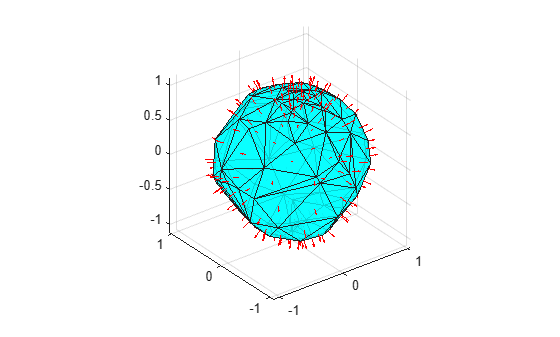faceNormal

Triangulation unit normal vectors

Description

F = faceNormal(TR) returns the unit normal vectors to all triangles in a 2-D triangulation. The faceNormal function supports 2-D triangulations only. F is a three-column matrix where each row contains the unit normal coordinates corresponding to a triangle in TR.ConnectivityList.

example

F = faceNormal(TR,ID) returns the unit normal vector to each triangle indexed by ID. The identification numbers of the triangles in TR are the corresponding row numbers of the property TR.ConnectivityList.

Examples

collapse all

Compute and plot the unit normal vectors to the facets of a triangulation on a spherical surface.

Create a set of points on a spherical surface.

rng default;
theta = rand([100,1])*2*pi;
phi = rand([100,1])*pi;
x = cos(theta).*sin(phi);
y = sin(theta).*sin(phi);
z = cos(phi);

Triangulate the sphere using the delaunayTriangulation function.

DT = delaunayTriangulation(x,y,z);

Find the free boundary facets of the triangulation, and use them to create a 2-D triangulation on the surface.

[T,Xb] = freeBoundary(DT);
TR = triangulation(T,Xb);

Compute the centers and face normals of each triangular facet in TR.

P = incenter(TR);
F = faceNormal(TR);

Plot the triangulation along with the centers and face normals.

trisurf(T,Xb(:,1),Xb(:,2),Xb(:,3), ...
'FaceColor','cyan','FaceAlpha',0.8);
axis equal
hold on
quiver3(P(:,1),P(:,2),P(:,3), ...
F(:,1),F(:,2),F(:,3),0.5,'color','r');Input Arguments

collapse all

Triangulation representation for 2-D triangulations only, specified as a scalar triangulation or delaunayTriangulation object.

Data Types: triangulation | delaunayTriangulation

Triangle identification, specified as a scalar or a column vector whose elements each correspond to a single triangle in the triangulation object. The identification number of each triangle is the corresponding row number of the ConnectivityList property.

Data Types: double